# Texas Go Math Grade 8 Lesson 1.3 Answer Key Ordering Real Numbers

Refer to our Texas Go Math Grade 8 Answer Key Pdf to score good marks in the exams. Test yourself by practicing the problems from Texas Go Math Grade 8 Lesson 1.3 Answer Key Ordering Real Numbers.

## Texas Go Math Grade 8 Lesson 1.3 Answer Key Ordering Real Numbers

Essential Question
How do you order a set of real numbers?

Reflect

Question 1.
If 7 + $$\sqrt{5}$$ is equal to $$\sqrt{5}$$ plus a number, what do you know about the number? Why?
We are told that 7 + $$\sqrt{5}$$ is equal to$$\sqrt{5}$$ plus a number. Lets denote with x the unknown number. We have:
7 + $$\sqrt{5}$$ = $$\sqrt{5}$$ + x
If we subtract $$\sqrt{5}$$ in both sides we get:
7 + $$\sqrt{5}$$ – $$\sqrt{5}$$ = $$\sqrt{5}$$ + x – $$\sqrt{5}$$
x = 7

The unknown number is 7 (whole number, integer, rational, real number).

Go Math Grade 8 Algebra Lesson 1.3 Question 2.
What are the closest two integers that $$\sqrt{300}$$ is between?
$$\sqrt{300}$$ Given
289 < 300 < 324 Find the perfect squares of the number that 300 is between

17 < $$\sqrt{300}$$ < 18 Take square roots
The close two integers are $17$ and $18$

Compare. Write <, >, or .

Question 3.
$$\sqrt{2}$$ + 4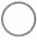2 + $$\sqrt{4}$$
First approximate $$\sqrt{2}$$ by comparing 2 to the closest perfect squares and then take square root from both sides of the equation.
1 < 2 < 4
$$\sqrt{1}$$ < $$\sqrt{2}$$ < $$\sqrt{4}$$ $$\sqrt{2}$$ is between 1 and 2. Next since 4 is a perfect square, we know that $$\sqrt{4}$$ = 2. $$\sqrt{2}$$ + 4 is between 5 and 6 2 + $$\sqrt{4}$$ is equal to 4. So, $$\sqrt{2}$$ + 4 > 2 + $$\sqrt{4}$$
$$\sqrt{2}$$ + 4 > 2 + $$\sqrt{2}$$

Question 4.
$$\sqrt{12}$$ + 612 + $$\sqrt{6}$$
$$\sqrt{12}$$ + 6 ? 12 + $$\sqrt{6}$$ Given
$$\sqrt{12}$$ is between 3 and 4 Approximate $$\sqrt{12}$$

$$\sqrt{6}$$ is between 2 and 3 Approximate $$\sqrt{6}$$

$$\sqrt{12}$$ + 6 is between 9 and 10 Use approximations to simply the expressions
12 + $$\sqrt{6}$$ is between 14 and 15
$$\sqrt{12}$$ + 6 < 12 + $$\sqrt{6}$$ Compare

Order the numbers from least to greatest. Then graph them on the number line.

Go Math Grade 8 Lesson 1.3 Independent Practice Answer Key Question 5.
$$\sqrt{5}$$, 2.5, $$\sqrt{3}$$ ____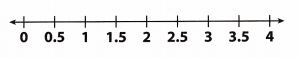$$\sqrt{5}$$, 2.5, $$\sqrt{3}$$ Given
4 < 5 < 9 Approximate $$\sqrt{5}$$. Find the perfect squares that 5 is between. Take square roots.
2 < $$\sqrt{5}$$ < 3
$$\sqrt{5}$$ is between 2 and 3
2.152 = 4.62 Find better estimates for $$\sqrt{5}$$ so it can be compared to 2.5.
2.22 = 4.84 5 is closer to 4,; try numbers between 2 and 2.5
2.252 = 5.06
Since $2.25^{2}$ is closer to $5$, an approximate value is $2.25$
1 < 3 < 4 Approximate $$\sqrt{3}$$. Find the perfect squares that 3 is between Take square roots.
1 < $$\sqrt{3}$$ < 2
$$\sqrt{3}$$ is between 1 and 2

1.62 = 2.56 Find better estimates for $$\sqrt{3}$$.
1.72 = 2.89 3 is closer to 4, hence try numbers between 1.5 and 2
1.82 = 3.24
Since $1.7^{2}$ is closer to $3$, an approximate value is $1.7$Graph on a Number line
$$\sqrt{3}$$, $$\sqrt{5}$$, 2.5 From the least to greatest

Math Talk
Mathematical Processes
If real numbers a, b, and c are in order from least to greatest, what is the order of their opposites from least to greatest? Explain.

Question 6.
π2, 10, $$\sqrt{75}$$ ____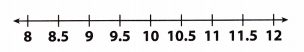π2, 10, $$\sqrt{75}$$ Given
64 < 75 < 81 Approximate $$\sqrt{75}$$ Find the perfect squares that 75 is between. Take square roots.
8 < $$\sqrt{75}$$ < 9
$$\sqrt{75}$$ is between 8 and 9

8.62 = 73.96 Find better estimates for $$\sqrt{75}$$
8.652 = 74.82 75 is closer to 81, hence try numbers between 8.5 and 9
8.78.62 = 75.69
Since $8.65^{2}$ is closer to $75$, an approximate value is $8.65$
π2 = (3.14)2 = 9.86 Find π2Graph on number line
$$\sqrt{75}$$, π, 10

Question 7.
Four people have found the distance in miles across a crater using different methods. Their results are given below.
Jonathan: $$\frac{10}{3}$$ Elaine: $$3 . \overline{45}$$, José: 3$$\frac{1}{2}$$, Lashonda: $$\sqrt{10}$$
Order the distances from greatest to least.
$$\frac{10}{3}$$ = $$3 . \overline{3}$$ ≈ 3.33 Express the given numbers as decimals.
$$3 . \overline{45}$$ = 3.454545 ≈ 3.45
3$$\frac{1}{2}$$ = 3.5
$$\sqrt{10}$$ is between 3.1 and 3.2. Since 3.22 = 10.24, an approximate value is 3.2.Graph on number line
3$$\frac{1}{2}$$ , $$3 . \overline{45}$$, $$\frac{10}{3}$$, $$\sqrt{10}$$ From greatest to least

Compare. Write <, >, or =. (Example 1)

Question 1.
$$\sqrt{3}$$ + 2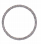$$\sqrt{3}$$ + 3
$$\sqrt{3}$$ + 2 ? $$\sqrt{3}$$ + 3
$$\sqrt{3}$$ is between 1 and 2 Approximate $$\sqrt{3}$$
$$\sqrt{3}$$ + 2 is between 3 and 4 Use approximations to simply the expressions
$$\sqrt{3}$$ + 3 is between 4 and 5
$$\sqrt{3}$$ + 2 < $$\sqrt{3}$$ + 3 Compare
$$\sqrt{3}$$ + 2 < $$\sqrt{3}$$ + 3

Go Math 8th Grade Compare and Order Real Numbers Answer Key Question 2.
$$\sqrt{11}$$ + 15$$\sqrt{8}$$ + 15.
First approximate $$\sqrt{11}$$ by comparing 11 to the closest perfect squares and then take square root from both sides of the equation
9 < 11 < 16
$$\sqrt{9}$$ < $$\sqrt{11}$$ < $$\sqrt{16}$$
3 < $$\sqrt{11}$$ < 4
$$\sqrt{11}$$ is between 3 and 4.
Next, approximate$$\sqrt{8}$$ by comparing 8 to the closest perfect squares and then take the square root from both sides of the equation.
4 < 8 < 9
$$\sqrt{4}$$ < $$\sqrt{8}$$ < $$\sqrt{9}$$
2 < $$\sqrt{8}$$ < 3 $$\sqrt{8}$$ is between 2 and 3.
$$\sqrt{11}$$ + 15 is then in between 18 and 19. $$\sqrt{8}$$ + 15 is between 17 and 18
Therefore, $$\sqrt{11}$$ + 15 > $$\sqrt{8}$$ + 15

$$\sqrt{11}$$ + 15 > $$\sqrt{8}$$ + 15

Practice and Homework Lesson 1.3 8th Grade Question 3.
$$\sqrt{6}$$ + 56 + $$\sqrt{5}$$
$$\sqrt{6}$$ + 5 ? 6 + $$\sqrt{5}$$ Given

4 < 6 < 9 Approximate $$\sqrt{6}$$
$$\sqrt{4}$$ < $$\sqrt{6}$$ < $$\sqrt{9}$$
2 < $$\sqrt{6}$$ < 3

4 < 5 < 9 Approximate $$\sqrt{5}$$
$$\sqrt{4}$$ < $$\sqrt{5}$$ < $$\sqrt{9}$$
2 < $$\sqrt{5}$$ < 3
$$\sqrt{6}$$ + 5 is between 7 and 8 Use approximately to simplify the expressions
6 + $$\sqrt{5}$$ is between 8 and 9
$$\sqrt{6}$$ + 5 < 6 + $$\sqrt{5}$$ Compare

Question 4.
$$\sqrt{9}$$ + 39 + $$\sqrt{3}$$
$$\sqrt{9}$$ + 3 ? 9 + $$\sqrt{3}$$
$$\sqrt{9}$$ = 3 Approximate $$\sqrt{9}$$
$$\sqrt{3}$$ is between 1 and 2 Approximate $$\sqrt{3}$$
$$\sqrt{9}$$ + 3 = 3 + 3 = 5 use approximations to simplify the expressions
9 + $$\sqrt{3}$$ is between 10 and 11
$$\sqrt{9}$$ + 3 < 9 + $$\sqrt{3}$$ Compare
$$\sqrt{9}$$ + 3 < 9 + $$\sqrt{3}$$

Question 5.
$$\sqrt{17}$$ – 3-2 + $$\sqrt{5}$$
$$\sqrt{17}$$ – 3 ? -2 + $$\sqrt{5}$$ Given
$$\sqrt{17}$$ is between 4 and 5 Approximate $$\sqrt{17}$$
$$\sqrt{5}$$ is between 2 and 3 Approximate $$\sqrt{5}$$

$$\sqrt{17}$$ – 3 is between 1 and 2 Use approximations to simplify the expressions
-2 + $$\sqrt{5}$$ is between 0 and 1
$$\sqrt{17}$$ – 3 > -2 + $$\sqrt{5}$$ Compare

Compare and Order Numbers Lesson 1.3 Question 6.
10 – $$\sqrt{8}$$12 – $$\sqrt{2}$$
10 – $$\sqrt{8}$$ ? 12 – $$\sqrt{2}$$ Given
$$\sqrt{2}$$ is between 1 and 2 Approximate $$\sqrt{8}$$
$$\sqrt{2}$$ is between 1 and 2 Approximate $$\sqrt{2}$$

10 – $$\sqrt{8}$$ is between 8 and 7 Use approximations to simplify the expressions
12 – $$\sqrt{2}$$ is between 11 and 10

10 – $$\sqrt{8}$$ < 12 – $$\sqrt{2}$$ Compare

Question 7.
$$\sqrt{7}$$ + 2$$\sqrt{10}$$ – 1.
$$\sqrt{7}$$ + 2 ? $$\sqrt{10}$$ – 1. Given
$$\sqrt{7}$$ is between 2 and 3 Approximate $$\sqrt{8}$$
$$\sqrt{10}$$ is between 3 and 4 Approximate $$\sqrt{10}$$

$$\sqrt{7}$$ + 2 is between 4 and 5 Use approximations to simplify the expressions
$$\sqrt{10}$$ – 1 is between 2 and 3

$$\sqrt{7}$$ + 2 > $$\sqrt{10}$$ – 1 Compare

Question 8.
$$\sqrt{17}$$ + 33 + $$\sqrt{11}$$
$$\sqrt{17}$$ + 3 ? 3 + $$\sqrt{11}$$ Given
$$\sqrt{17}$$ is between 4 and 5 Approximate $$\sqrt{17}$$
$$\sqrt{11}$$ is between 3 and 4 Approximate $$\sqrt{11}$$

$$\sqrt{17}$$ + 3 is between 7 and 8 Use approximations to simplify the expressions
3 + $$\sqrt{11}$$ is between 6 and 7

$$\sqrt{7}$$ + 3 > 3 + $$\sqrt{11}$$ Compare

Order $$\sqrt{3}$$, 2π, and 1.5 from least to greatest. Then graph them on the number line. (Example 2)
$$\sqrt{3}$$ is between ___ and ___, so $$\sqrt{3}$$ ≈ ____.
π ≈ 3.14, so 2π ≈ ____.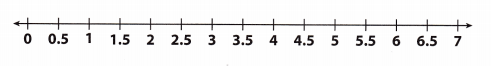From least to greatest, the numbers are ____ , _____
______
$$\sqrt{3}$$ is between 1.7 and 1.8 since 1.72 = 2.89, a good approximation for $$\sqrt{3}$$ is 1.7.
1.5 is in good form so we don’t need to change it.
π ≈ 3.14 ⇒ 2π ≈ 6.28

Graphing:
A = 1.7, B = 1.5 and C = 6.28.From least to greatest, the numbers are 1.5, $$\sqrt{3}$$, 2π

From least to greatest, the numbers are 1.5, $$\sqrt{3}$$, 2π. Click for the graph.

Question 10.
Four people have found the perimeter of a forest using different methods. Their results are given in the table. Order their calculations from greatest to least. (Example 3)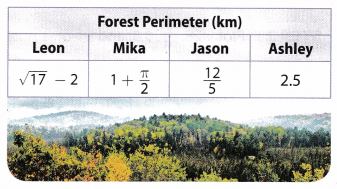$$\sqrt{3}$$ – 2 Estimate the value of $$\sqrt{3}$$
$$\sqrt{3}$$ is between 4 and 5
Since, $17$ is closer to $16$, the estimated value is $4.1$
1 + $$\frac{\pi}{2}$$ Where π = 3.14
1 + $$\frac{3.14}{2}$$ = 2.57

$$\frac{12}{5}$$ = 2.4 Evaluate the fraction
2.5 Given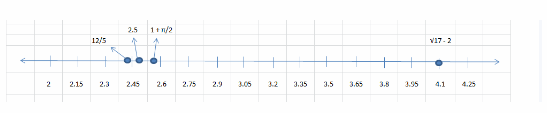Graph on number line
$$\sqrt{17}$$ – 2, 1 + $$\frac{\pi}{2}$$, 2.5, $$\frac{12}{5}$$ From greatest to least

Essential Question Check-in

Question 11.
Explain how to order a set of real numbers.
To order real numbers first express them in the decimal form. If all numbers can’t be calculated to a finite decimal,
approximate them to a chosen accuracy (number of digits behind the decimal point, where more digits equal higher accuracy). Then you can easily place them on a number line.

Express numbers in decimal form and approximate.

Order the numbers from least to greatest.

Question 12.
$$\sqrt{7}$$, 2, $$\frac{\sqrt{8}}{2}$$
$$\sqrt{7}$$, 2, $$\frac{\sqrt{8}}{2}$$ Given
$$\sqrt{7}$$ Estimate the value of $$\sqrt{7}$$
$$\sqrt{7}$$ is between 2 and 3
Since, $7$ is closer to $9$, $2.65^{2} = 7.02$, hence the estimated value is $2.65$

2 Given

$$\frac{\sqrt{8}}{2}$$ Estimate the value of $$\sqrt{8}$$ and simplify
$$\sqrt{8}$$ is between 2 and 3
Since, $8$ is closer to $9$, $2.85^{2} = 8.12$, hence the estimated value is $2.85$
$$\frac{2.85}{2}$$ = 1.43Graph on a number line
$$\frac{\sqrt{8}}{2}$$, 2, $$\sqrt{7}$$ From least to greatest

$$\sqrt{10}$$, π, 3.5
$$\sqrt{10}$$ is between 3.1 and 3.2. In some cases, this approximation is precise enough, but this is not the case. Since we have a given number π ≈ 3.14 that ¡s also between 3.1 and 3.2 we must be more precise. So, an even better approximation is 3.15 because 3.152 ≈ 9.92.
π ≈ 3.14

We can now order the numbers from [east to greatest easily:
Because 3.14 < 3.15 < 3.5 it follows that π < $$\sqrt{10}$$ < 3.5
Numbers from least to greatest π < $$\sqrt{10}$$ < 3.5

Question 14.
$$\sqrt{220}$$, -10, $$\sqrt{100}$$, 11.5
$$\sqrt{220}$$, -10, $$\sqrt{100}$$, 11.5 Given
196 < 220 < 225 Approximate $$\sqrt{220}$$
$$\sqrt{196}$$ < $$\sqrt{220}$$ < $$\sqrt{225}$$
14 < $$\sqrt{220}$$ < 15
$$\sqrt{220}$$ ≈ 14.5
$$\sqrt{100}$$ = 10 Calculate $$\sqrt{100}$$Plot on a number line
-10, $$\sqrt{100}$$, 11.5, $$\sqrt{220}$$ Order from least to greatest
-10, $$\sqrt{100}$$, 11.5, $$\sqrt{220}$$

Question 15.
$$\sqrt{8}$$, -3.75, 3, $$\frac{9}{4}$$
$$\sqrt{8}$$, -3.75, 3, $$\frac{9}{4}$$ Given
$$\sqrt{8}$$ Estimate the value of $$\sqrt{8}$$
$$\sqrt{8}$$ is between 2 and 3
Since, $8$ is closer to $9$, $2.85^{2} = 8.12$, hence the estimated value is $2.85$
-3.75 Given
3
$$\frac{9}{4}$$ = 2.25 Evaluate the fraction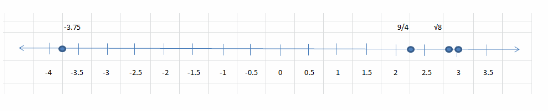Graph on the number line
-3.75, $$\frac{9}{4}$$, $$\sqrt{8}$$, 3 From least to greatest

Ordering Real Numbers 8th Grade Question 16.
Your sister is considering two different shapes for her garden. One is a square with side lengths of 3.5 meters, and the other is a circle with a diameter of 4 meters.
a. Find the area of the square. _______
To find the area of a square use the formula: A = x2 where x is the side of the square and the area is represented by A.
So, A = 352 = 12.25

To find the area of a square use the formula: B = r2π where r is the radius of the circle and the area is represented by B.
So, B = 22π = 12.57 (Diameter d is twice the radius r, so r = 2.)

The garden shaped like a circle would give her more space to plant because it has a bigger area.
A = 3.52 = 12.25

b. Find the area of the circle. ____
B = 22π = 12.57

c. Compare your answers from parts a and b. Which garden would give your sister the most space to plant?
The circle-shaped garden has more space to plant.

Question 17.
Winnie measured the length of her father’s ranch four times and got four different distances. Her measurements are shown in the table.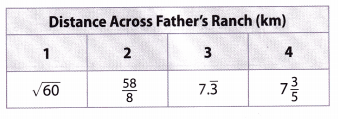a. To estimate the actual length, Winnie first approximated each distance to the nearest hundredth. Then she averaged the four numbers. Using a calculator, find Winnie’s estimate.
$$\sqrt{60}$$ ≈ 7.75 Evaluate the lengths to nearest hundredth (using calculator)
$$\frac{58}{8}$$ ≈ 7.25
$$7 . \overline{3}$$ ≈ 7.33
7$$\frac{3}{5}$$ ≈ 7.60
Average = $\dfrac{7.75 + 7.25 + 7.33 + 7.60}{4} = 7.4815$

b. Winnie’s father estimated the distance across his ranch to be $$\sqrt{56}$$ km. How does this distance compare to Winnie’s estimate?
$$\sqrt{56}$$ ≈ 7.4833 Using claculator
They are nearly identical

Give an example of each type of number.

Question 18.
a real number between $$\sqrt{13}$$ and $$\sqrt{14}$$ _____
Since, $$\sqrt{13}$$ ≈ 3.61 < 3.7 < $$\sqrt{14}$$ ≈ 3.74
3.7 is a good example of a real. number that is in between $$\sqrt{13}$$ and $$\sqrt{14}$$

3.7

Question 19.
an irrational number between 5 and 7 _________
Square the given numbers to find their perfect squares and then a square root of any number in between those two is an example of an irrational number between 5 and 7:
52 = 25
72 = 49
So, one example is $$\sqrt{31}$$
$$\sqrt{31}$$

Question 20.
A teacher asks his students to write the numbers shown from least to greatest. Paul thinks the numbers are already in order. Sandra thinks the order should be reversed. Who is right?Approximate every given number in decimal form:
Use dosest perfect squares to approximate
100 < 115 < 121
$$\sqrt{100}$$ < $$\sqrt{115}$$ < $$\sqrt{121}$$
10 < $$\sqrt{115}$$ < 11
A good approximation for $$\sqrt{115}$$ is 10.7 since 10.72 = 114.5
$$\frac{115}{11}$$ ≈ 10.45
Finally, that gives us the approximated numbers that the teacher gave them in the task: 10.7, 10.45, 10.5624
We can conclude that neither is right because they are not in any order

Neither are right The numbers aren’t in any order.

Question 21.
Math History There is a famous irrational number called Euler’s number, often symbolized with an e. Like π, it never seems to end. The first few digits of e are 2.7182818284.

a. Between which two square roots of integers could you find this number?
2.718281828
The square of e lies between 7 and 8
2.722 = 7.3984
Hence, it lies between $\sqrt{7} = 2.65$ and $\sqrt{8} = 2.82$

b. Between which two square roots of integers can you find π?
The square of π lies between 9 and 10
3.142
3.142 = 9.8596
Hence, it lies between $\sqrt{9} = 3$
and $\sqrt{1ø} = 3.16$

Texas Go Math Grade 8 Lesson 1.3 H.O.T. Focus On Higher Order Thinking Answer Key

Question 22.
Analyze Relationships There are several approximations used for π, including 3.14 and $$\frac{22}{7}$$.π is approximately 3.14159265358979…

a. Label π and the two approximations on the number line.a) We plot on number line π, 3.14 and $$\frac{22}{7}$$: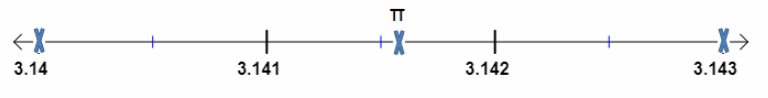b. Which of the two approximations is a better estimate for π? Explain.
As we can see from the number line, $$\frac{22}{7}$$ is closer to π, so we can conclude that $$\frac{22}{7}$$ is a better estimation for π.

c. Find a whole number x in $$\frac{x}{113}$$ so that the ratio is a better estimate for π than the two given approximations. ______
$$\frac{355}{113}$$ is a better estimation for π, because
$$\frac{355}{113}$$ = 3.14159292035 ≈ 3.14159265358979 = π

Go Math 8th Grade Lesson 1.3 Compare and Order Real Numbers Question 23.
Communicate Mathematical Ideas If a set of six numbers that include both rational and irrational numbers is graphed on a number line, what is the fewest number of distinct points that need to be graphed? Explain.
The fewest number of distinct points that need to be graphed is 2. Because there are both rational and irrational
numbers in the set and every rational number is not irrational and every irrational is not rational they can’t all be the same.
Therefore it could be that there are some numbers that repeat but there are at least two different numbers.

Two. Click for proof.

Question 24.
Critique Reasoning Jill says that $$12 . \overline{6}$$ is less than 12.63. Explain her error.
$$12 . \overline{6}$$ = 12.666666….
If we round that number to two decimal Places we have $$12 . \overline{6}$$ ≈ 12.67.